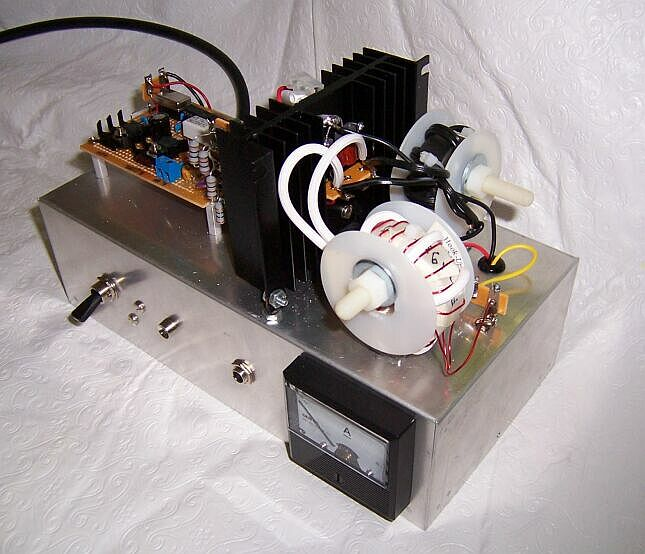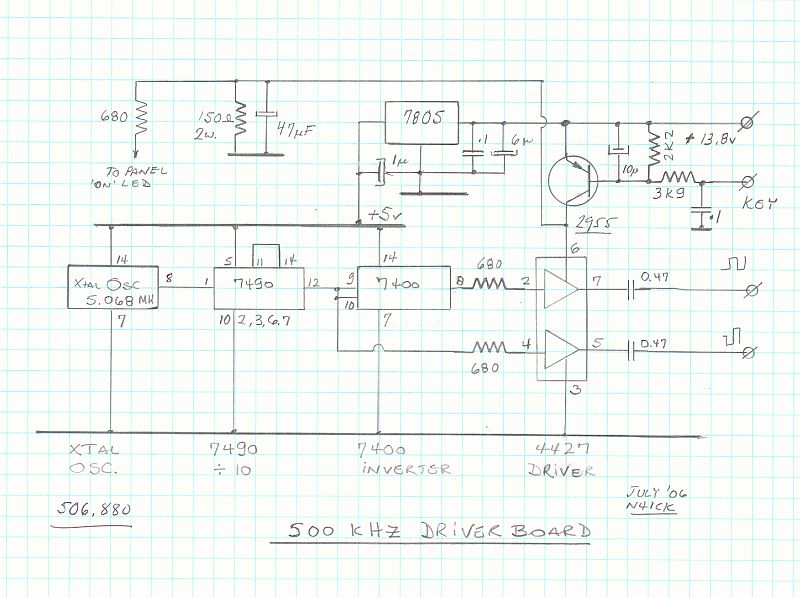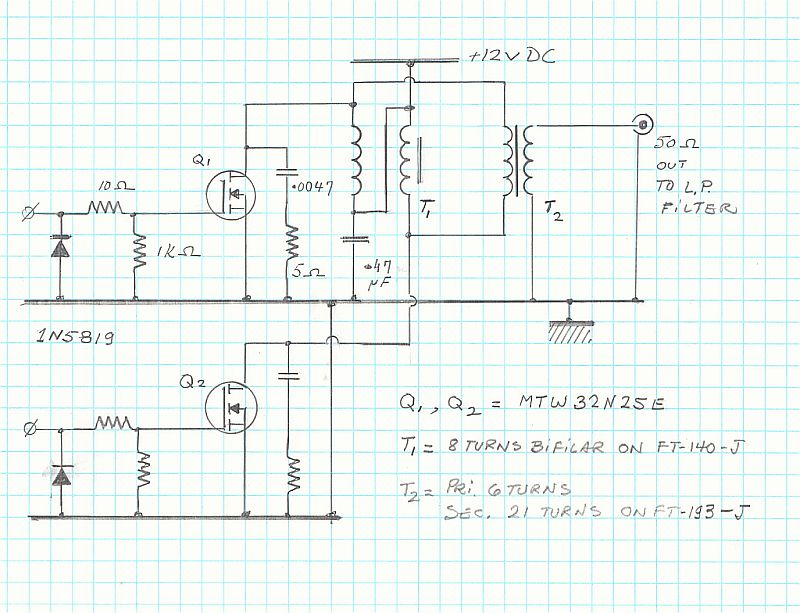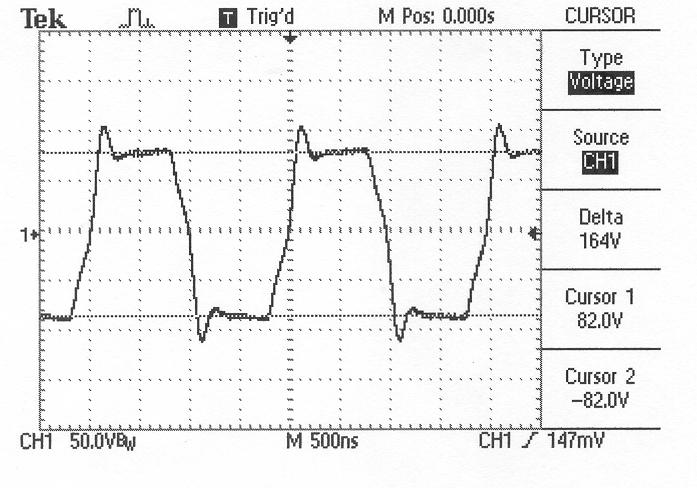25-02-2011 23:37

--
António Matias

# A 100 Watt CW Transmitter for the 500 kHz Band

André Kesteloot N4ICK
16 July 2006The output of a standard "can" Xtal oscillator at 5,068 kHz is fed to a 7490 divide-by-10 stage. The resulting 506.8 kHz square wave is fed directly to ½ of the 4427 driver, and also inverted by ¼ of the 7400 that feeds the other half of the 4427 (Q and not Q).

The Q (and not Q) square-wave is first DC-restored by the 1N5819 Schottky diode and then applied to the gate of a MTW32N25E power MOSFET. A Zobel network consisting of a 5 Ohm resistor and a 4.7nF polypropylene capacitor was inserted at the drain to avoid potential problems.

The two power MOSFETS are parallel-fed via T1, while T2 matches the low output impedance of these MOSFETs to a 50 Ohm load.

Testing was conducted into a 50 Ohm dummy load.

The output waveform is a reasonable-looking square-wave, 184 volts Peak-to-Peak.

Power input : 12 volts DC at 12 amperes = 144 watts.

Power output: 134 watts square wave (in fact a little less, as the square wave is not perfect).

Efficiency = 134/144 = 93%

Theoretical fundamental content = [4 * 82/p * sin x ] = 104 watts Output power after a two stage low-pass filter = about 99 watts (to be further optimized).

The MTW32N25E MOSFETs have the following specifications:

32 amps; 250 volts; Ron = 0.07 Ohm; PD = 250 watts @ 25°C.;

(This design is based on several transmitters previously built for operation in the 136 kHz band. See, for instance, RadCom, February 2003, page 67).

N4ICKCT1FFU - CR5A

QRV: HF, 6m, 4m,2m,70cm,23cm
SSB, CW, MGM
DX, EME, Contest,Sat.

Fonte: ct-comunicacoes-e-tecnologias@googlegroups.com Em nome de Antonio Matias